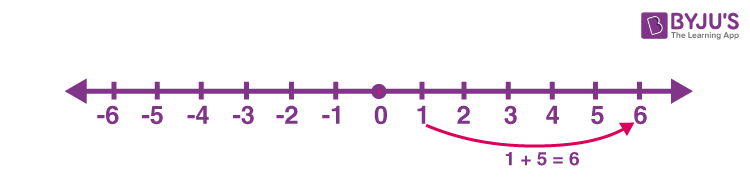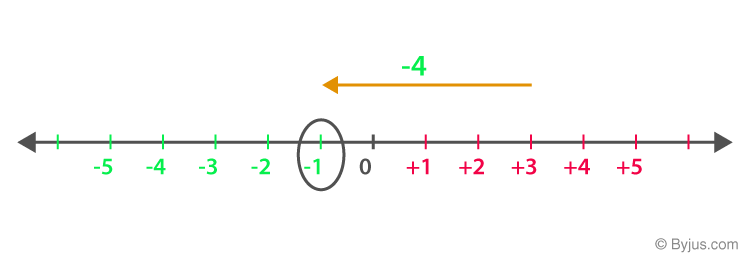Addition and subtraction are the two primary arithmetic operations where we learn to add and subtract two or more numbers or any mathematical values. The other two basic math operations are multiplication and division. The symbol to represent addition is ‘+’ (plus sign) and subtraction is ‘-’ (minus sign).

Both addition and subtraction are inverse operations of each other. For example, if 9 + 1 = 10, then 10 – 1 = 9. That shows if 1 is added to 9 then the result is 10, whereas if 1 is subtracted from 10, then the result is 9.

Addition means summing up two or more numbers or values to get another number. For example, if we add 2 and 3, we get 5 as the result.

2 + 3 = 5

Now, let us understand the sum of 2 and 3 practically. Suppose, we have 2 apples in a basket and 3 more apples are added in the same basket, so how many apples are there altogether?

2 → 1 + 1

3 → 1 + 1 + 1

2 + 3 = 1 + 1 + 1 + 1 + 1

Now if we count each apple, then it is equal to 5.

The property of addition is to increase the value by adding another value to it.

## What is Subtraction?

Subtraction means to reduce a value from another value to get the required value.

For example, 3 subtracted from 5 we get 2 as the answer.

5 – 3 = 2

Now, if we imagine, there are 5 apples in a basket and we took out 3 apples from the basket, then how many apples are left?

5 – 3 = 1 + 1 + 1 + 1 + 1 – 1 – 1 – 1 = 2

## Addition, Subtraction, Multiplication and Division

As we mentioned earlier, the four major operations in mathematics are:

• Subtraction
• Multiplication
• Division

Multiplication and division are also the inverse operations of each other.

• 2 multiplied by 3 is 6
• 6 divided by 3 is 2

Also, multiplication of numbers, displays the repeated addition.

• 2 x 4 = 8
• 2 + 2 + 2 + 2 = 8

Learn Order of Operations-BODMAS Rule here at BYJU’S.

## Rules

The rules to add and subtract numbers are given below:

• Addition of two positive numbers is always positive
• Addition of two negative numbers is always negative
• Subtraction of two positive numbers can be either positive or negative
• Subtraction of two negative numbers can be either positive or negative
 Positive + Positive Addition (Sign will be Positive) 3 + 4 = 7 Negative + Negative Addition (Sign will be negative) – 3 + (-4) = -7 Positive + Negative Subtraction (Sign of greater number) 3 + (-4) = -1 Positive – Negative Addition (- x – = +) 3 – (-4) = 3 + 4 = 7 Negative – Positive Addition -3 – (4) = -7

Check rules for addition and subtraction of decimals.

## For One Digit

One digit numbers are all the single-digit integers, i.e.

 1 2 3 4 5 6 7 8 9 -1 -2 -3 -4 -5 -6 -7 -8 -9

If we add any two one-digit positive numbers, then the result is either a single-digit or double-digit number.

1+7 = 8

8 + 7 = 15

### Subtraction of one-digit numbers

Subtraction of any two one-digit positive numbers will always result in a single-digit number.

8 – 5 = 3

9 – 1 = 8

## For Two digit numbers

We can take two-digit positive and negative numbers to add and subtract.

Addition of two-digit positive numbers may result in a 3-digit value but subtraction of two digit positive numbers will not result in a three-digit value. A few examples are:

• 22 + 13 = 35
• 99 + 10 = 109
• 99 – 10 = 89
• 80 – 15 = 65

## For Three digit numbers

In the case of three-digit positive numbers, the same rules apply for addition and subtraction that are similar to one-digit and two-digit numbers.

• 100+101 = 201
• 999 + 100 = 1099
• 100 – 1 = 99
• 999 – 100 = 899

## Addition and subtraction of Fractions

Fractions are the value in Maths, that represents part of a whole. Examples of fractions are ½, ⅓, ¼, ¾, ⅔, etc.

½ + ½ = 1

It shows if two halves are added together, then it results in a whole.

Subtracting fractions:

¾ – ¼ = 2/4 = ½

Learn how to perform addition and subtraction of fractions here.

## Addition and subtraction with regrouping

Regrouping, in addition, is done if the sum of numbers in the unit place will result in a number more than 9, and the additional digit is carried forward to the next value at ten’s place and gets added. Let us see an example to understand.

21 41 8

1 7 5

————

4 2 3

————

In the above example, 8 + 5 = 13, thus we kept 3 at the unit place of the answer, and 1 is carried forward and added to 4 and 7. Now, 1+4+7 = 12. So, 2 is kept and 1 is again carried forward to add with 2 and 1. 1+2+1 = 4.

Regrouping for subtraction:

Subtract 182 from 427.

4 2 7

– 1 8 2

————–

2 4 5

————–

In the above example, at the unit place, 7 – 2 gives 5, but at ten’s place, we need to borrow 1 to group 2 as 12 and then subtract 8 from it. So, 12 – 8 = 4. Since 1 is taken from 4 at a hundred’s place, thus we are left with 4 -1 = 3. Now subtract 3 – 1 = 2.

## Worksheets

Students of Class 2, Class 3, Class 4 and Class 5 can practise the addition and subtraction of numbers with the help of these worksheets. These questions will help them to brush up their skills in this concept.

1. 6 – (-9) = ?

2. 10 – (10) = ?

3. 10 – (8) = ?

4. 34 – (-9) = ?

5. 73 – (88) = ?

6. 78+ (-12) = ?

7. 68 + (-56) = ?

8. 36 + (9) = ?

9. 94 + (-99) = ?

10: (-63) + (0) = ?

## Word problems

The word problems based on addition and subtraction are given below along with solutions.

Q.1: Ritu has 7 balloons and her friend Seema has 5 balloons. How many more balloons do Ritu have than her friend?

Solution: Given, we have 7 balloons and our friend has 5 balloons.

7 – 5 = 2

Thus, Ritu has 2 more balloons than her friend.

Q.2: 114 birds were sitting on a tree. 21 more birds flew up to the tree. How many birds were there altogether on the tree?

Solution: Given, there are 114 birds on a tree.

21 more birds flew up to the tree.

114 + 21 = 135

There were 135 birds on the tree.

### Addition and Subtraction on Number line

The addition of numbers on the number line can be done by moving the number of steps as the number to be added towards the right of 0.

Addition of 1 and 5 is represented using the number line as:Thus, 1 + 5 = 6

Incase of subtraction, we need to move towards the left of 0.

For example, subtraction of 4 from 3 can be represented as shown in the below figure.Therefore, 3 – 4 = -1

### Practice Problems

1. Add 72, 56 and 29.
2. Subtract 120 from 199.
3. What is the value of -58 + (-47)?
4. Evaluate: 69 – (-34)

### What is addition and subtraction?

Addition and subtraction are two primary arithmetic operations, where we find the sum and difference of two numbers. Addition is denoted by ‘+’ and subtraction by ‘-’. Both are inverse operations of each other.

Example: 5 + 6 = 11 and 11 – 6 = 5

### What are the rules of addition?

When we add two positive numbers, it results in positive value. Adding two negative numbers, results in the sum of the two numbers but with a negative sign. If we add two numbers with opposite signs, then it results in subtraction.

### What are the rules of subtraction?

Rules of subtraction with examples are:

12 – 2 = 10

-12 – (-2) = -12 + 2 = -10

### What is the value of 9 – 2 + 1?

9 – 2 + 1

First we will add 9 and 1

10 – 2

Now subtract.

8